# please help with all parts a. b. c. d. What are the conditions on L1 and...

a.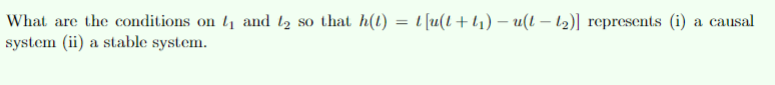b.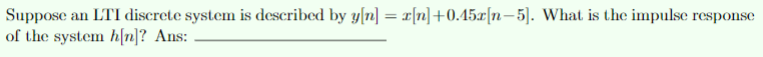c.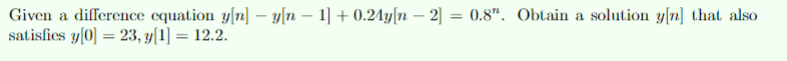d.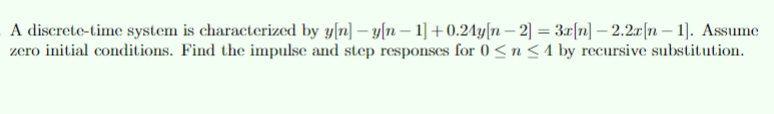What are the conditions on L1 and 12 so that h(I) = 1 [u(L+1) -ul- 12) represents (i) a causal system (ii) a stable system.
[n] +0.15.(n-5). What is the impulse response Suppose an LTI discrete system is described by y[n] = of the system h[n]? Ans: -
Given a difference equation y[n] - y(n - 1] +0.24y[n - 2) = 0.8". Obtain a solution y[n] that also satisfies y0 = 23, y1 = 12.2.
- A discrete-time system is characterized by y[n] - y[n – 1] +0.24y[n - 2) = 3.[n] - 2.22 [n – 1]. Assume zero initial conditions. Find the impulse and step responses for 0 <n<1 by recursive substitution.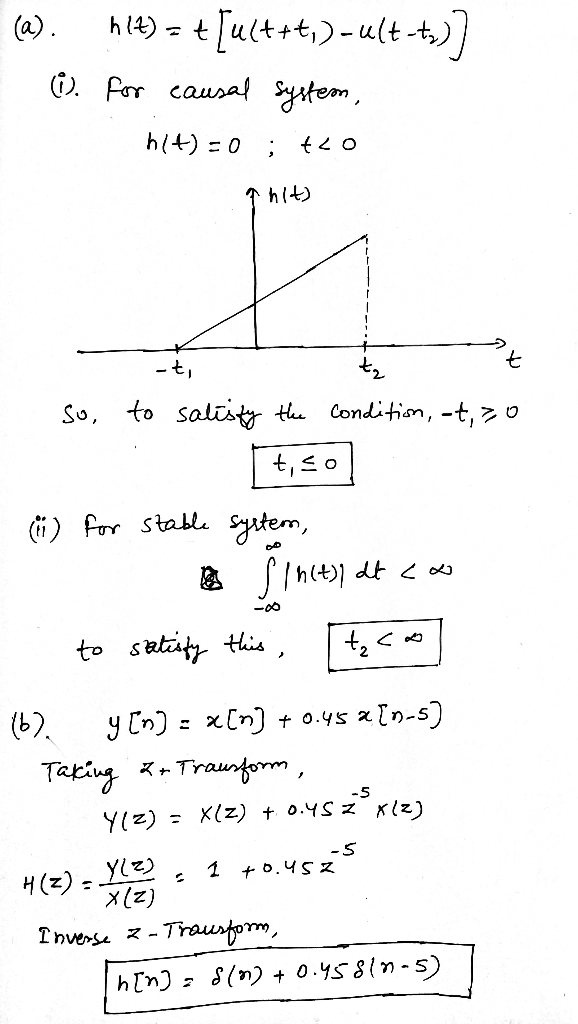#### Earn Coin

Coins can be redeemed for fabulous gifts.

Similar Homework Help Questions
• ### Could you please help with the following parts: a. b. c. A system has the impulse response of. If the following signal is input into this system: Enter the output of this system (y[지) into the bla...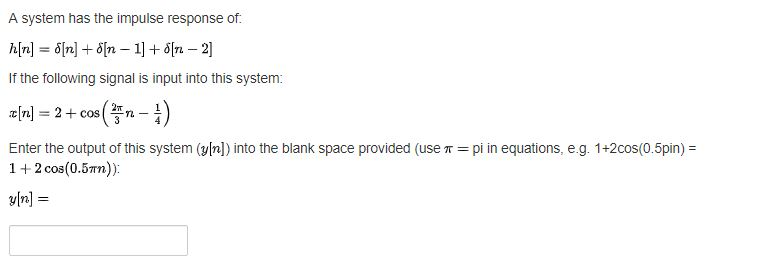Could you please help with the following parts: a. b. c. A system has the impulse response of. If the following signal is input into this system: Enter the output of this system (y[지) into the blank space provided (use π = pi in equations, eg. 1 +2cos(0.5pin) = 12 cos(0.5Tn)) The magnitude response of a discrete-time filter is plotted between the range-4T ώ +6x and is shown below. 10 -2 0 Normalised Radian frequency x Tm What type of...

• ### 4. (5 pts) Consider a discrete-time LTI system T that generates an output y[n] according to...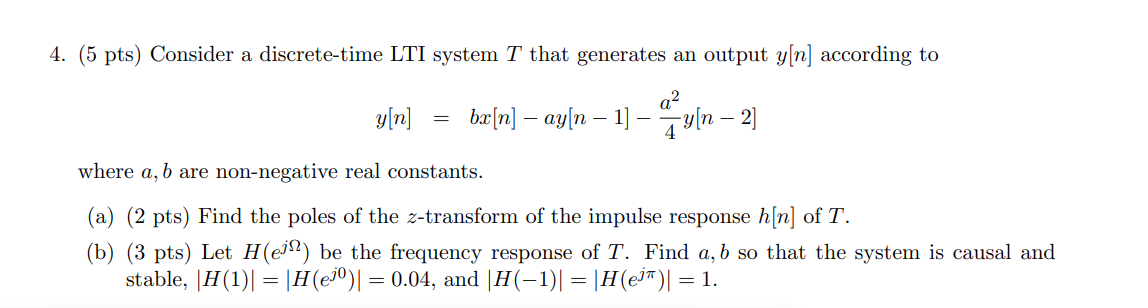4. (5 pts) Consider a discrete-time LTI system T that generates an output y[n] according to a2 y[n] bx[n] – ay[n – 1] - *[n – 2] where a, b are non-negative real constants. (a) (2 pts) Find the poles of the z-transform of the impulse response h[n] of T. (b) (3 pts) Let H(ejl be the frequency response of T. Find a, b so that the system is causal and stable, |H(1)| = |H(ejº)] = 0.04, and |H(-1)] =...

• ### Please just answer a, b, c Question #8: (5 pts) The voltage impulse response of an...Please just answer a, b, c Question #8: (5 pts) The voltage impulse response of an RC circuit is h(t) 2t e'"u(t = (a) Is the LTI system defined by h(t) causal? Why? (b) Is the LTI system defined by h(t) memoryless? Why? (c) Is the LTI system defined by h(t) BIBO stable? Why? (d) Compute the step response (the charging response) of the system y(t)ut) h(t) (e) Compute the response of the system y(t)-x(t) *h(t) with input r(t) e-tu(t).

• ### 5. (20 points) For a causal LTI discrete-time system described by the difference equation: y[n] +...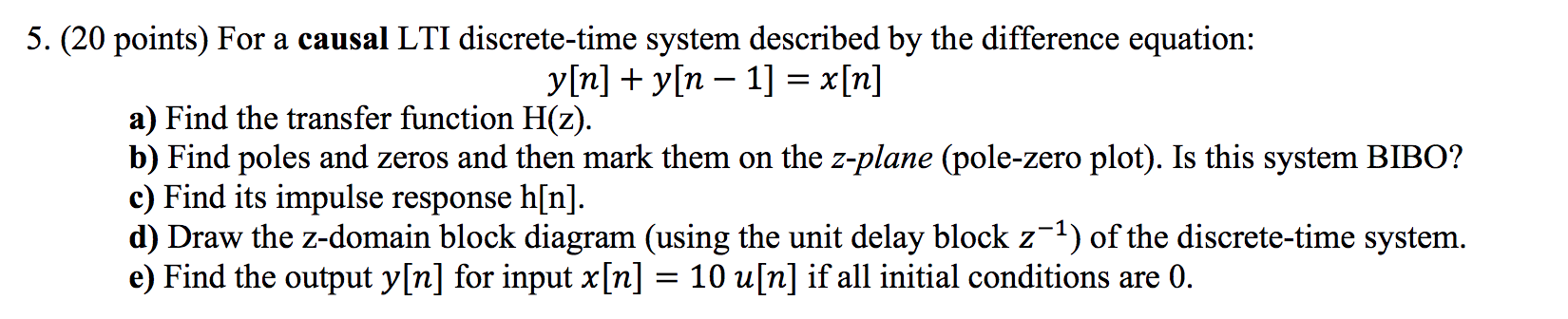5. (20 points) For a causal LTI discrete-time system described by the difference equation: y[n] + y[n – 1] = x[n] a) Find the transfer function H(z). b) Find poles and zeros and then mark them on the z-plane (pole-zero plot). Is this system BIBO? c) Find its impulse response h[n]. d) Draw the z-domain block diagram (using the unit delay block z-1) of the discrete-time system. e) Find the output y[n] for input x[n] = 10 u[n] if all...

• ### QUESTION 2 (20 MARKS) (a) A continuous time signal x(t) = 3e2tu(-t) is an input to...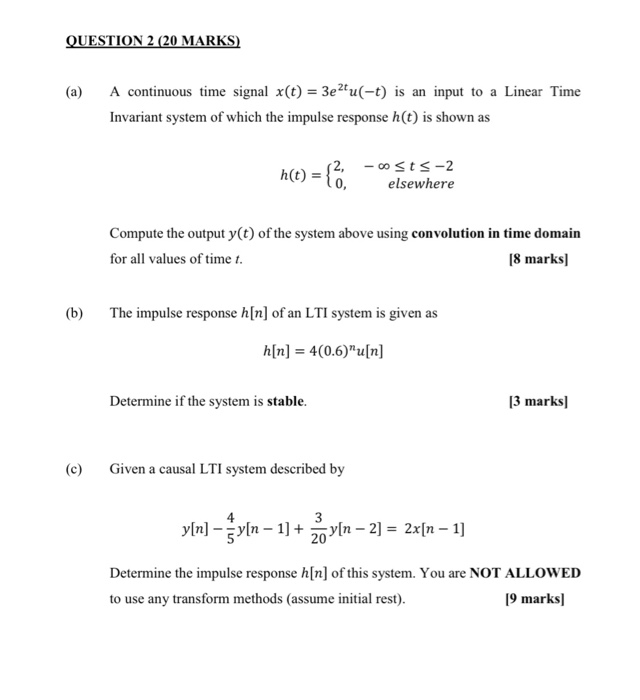QUESTION 2 (20 MARKS) (a) A continuous time signal x(t) = 3e2tu(-t) is an input to a Linear Time Invariant system of which the impulse response h(t) is shown as h(t) = { .. 12, -osts-2 elsewhere Compute the output y(t) of the system above using convolution in time domain for all values of time t. [8 marks) (b) The impulse response h[n] of an LTI system is given as a[n] = 4(0.6)”u[n] Determine if the system is stable. [3...

• ### Will thumbs up if completed. Please show all work and have clear images, thank you. 5....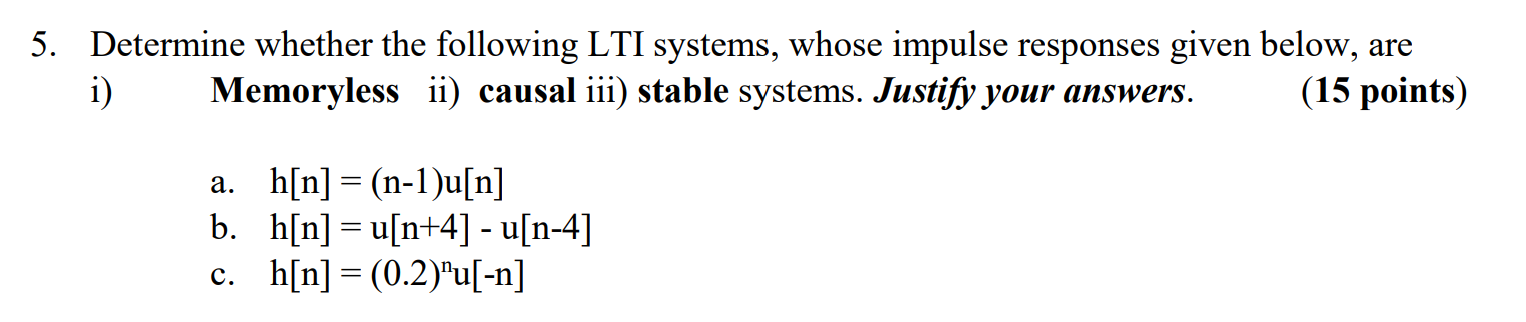Will thumbs up if completed. Please show all work and have clear images, thank you. 5. Determine whether the following LTI systems, whose impulse responses given below, are i) Memoryless ii) causal iii) stable systems. Justify your answers. (15 points) a. h[n] =(n-1)u[n] b. h[n]=a[n+4] - u[n-4] c. h[n] = (0.2)"u[-n]

• ### QUESTION 4 a. Determine the frequency spectrum of the signal x(t) b. What is the Nyquist...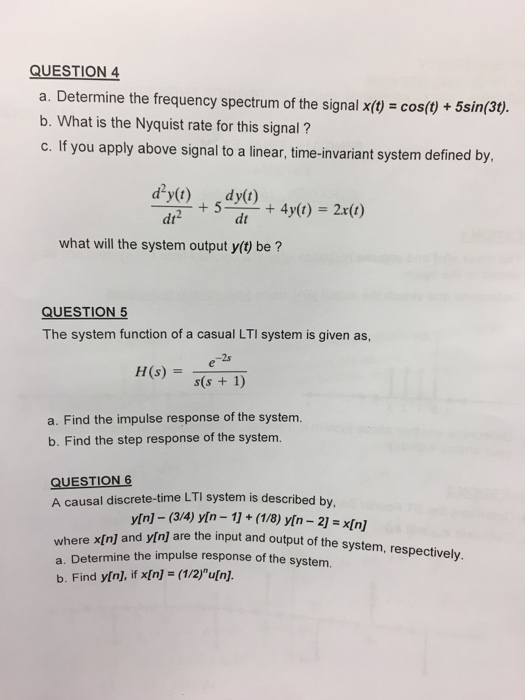QUESTION 4 a. Determine the frequency spectrum of the signal x(t) b. What is the Nyquist rate for this signal? cos(t) + 5sn3t. me-invariant system defined by, dt dt what will the system output y(t) be? QUESTION 5 The system function of a casual LTI system is given as, 2s a. Find the impulse response of the system. b. Find the step response of the system. A causal discrete-time LTI system is described by, y[n] - (3/4) yin-1+(1/8) yin-21 xn]...

• ### 2. A discrete time LTI system is described by the difference equation (assume initial conditions are...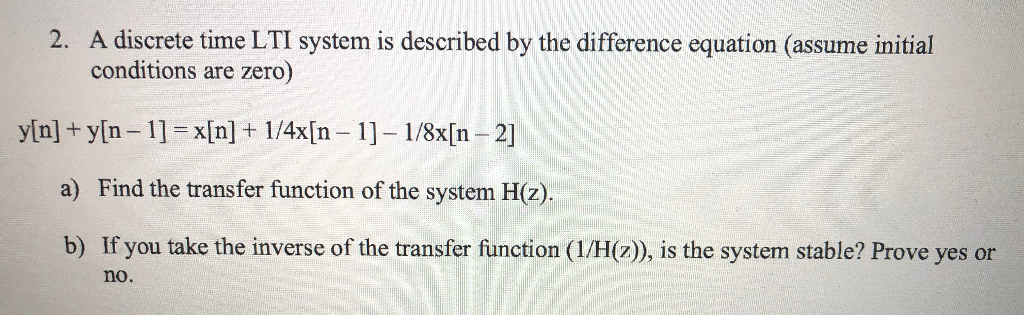2. A discrete time LTI system is described by the difference equation (assume initial conditions are zero) y[n] + y[n – 1] = x[n] + 1/4x[n – 1] – 1/8x[n – 2] a) Find the transfer function of the system H(z). b) If you take the inverse of the transfer function (1/H(z)), is the system stable? Prove yes or no.

• ### please answer all questions with working out! will rate high A signal : = cos( /4)0.8"...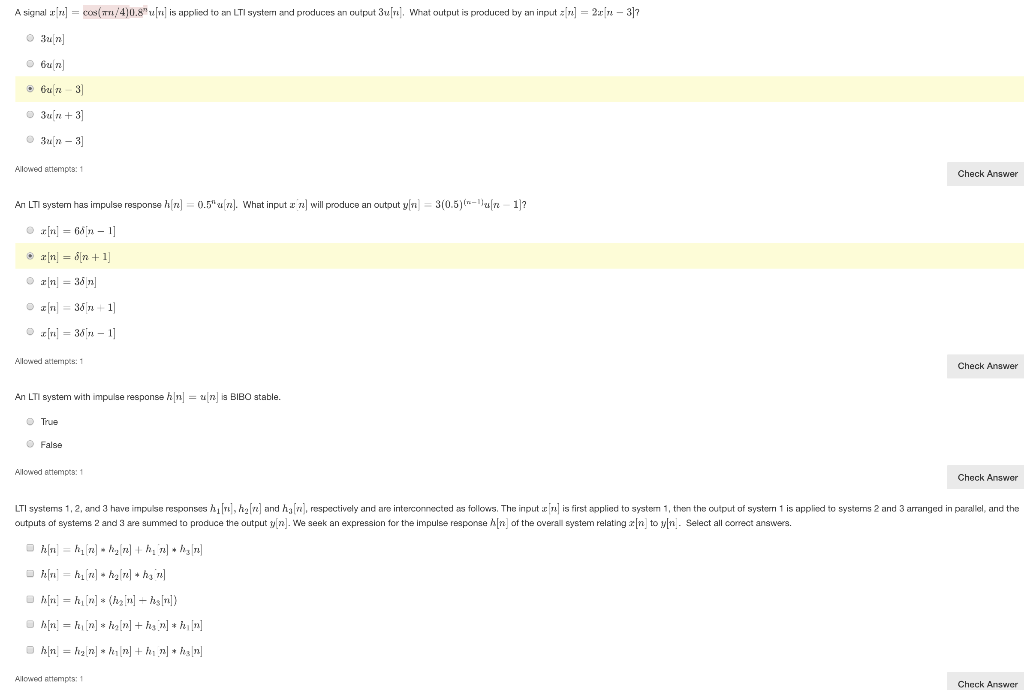please answer all questions with working out! will rate high A signal : = cos( /4)0.8" [rel is applied to an LTI systern and produces an output 3u. What output is produced by an input airl] = 2:0[– 317 bur un 3 3un +3] 3un - 3] Allowed attempts: 1 Check Answer An LTI system has impulse response h[n] = 0.5" (n). What input : 1) will produce an output y(n) = 3(0.5)(n-Halle 1)? [n] = 68 n - 1]...

• ### please show detailed work/proof 3. The input and output of a causal LTI system satisfy the...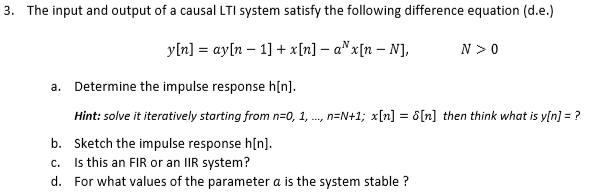please show detailed work/proof 3. The input and output of a causal LTI system satisfy the following difference equation (d.e.) y[n] = ayln-1] + x[n]-a"x[n-N], N > 0 a. Determine the impulse response h[n]. Hint: solve it iteratively starting from n=0, 1, , n=N+1; x[n] = δ[n] then think what is y[n] ? b. Sketch the impulse response h[n] c. Is this an FIR or an IIR system? d. For what values of the parameter a is the system stable?